# Distances on Earth 3: Planar Approximation

We’ve looked at two formulas for the distance between points given their latitude and longitude; here we’ll examine one more formula, which is valid only for small distances. This is a “flat-earth approximation” to distance.

## Distances within ten meters?

The first question is from 2002:

Transformation between (x,y) and (longitude, latitude)

Dear Dr. Math,

I have two questions on the transformation between (x,y) and (longitude, latitude).

1. Given 2 positions in terms of longitude and latitude, say, (a1, b1) and (a2,b2) with the distance between them very small, say, within 10 meters, I would like to know if there are any methods that can compute their separation with the best accuracy. I converted the (a,b) to (x,y) using the following formulae:

x = R*cos(a)*cos(b)
y = R*cos(a)*sin(b)

where R is the earth radius. The distance is calculated as the square root of (x1-x2)^2+(y1-y2)^2. Please comment on my method.

2. I have a digital map giving the four corners in terms of longitudes and latitudes, say, (a1,b1), (a1,b2), (a2,b1), (a2,b2). I would like to know how to compute the (x,y) of a particular point in the map.

Thank you very much.

Hing is apparently thinking that transforming coordinates on earth to $$(x,y)$$ coordinates on a map will allow reasonably accurate distance calculations on the map; that’s true, but the reality is that there are different map “projections” that might be used on his map, and his formula is not appropriate for a map anyway. We’ll be focusing on a particular projection that is appropriate for short-range distance calculations, rather than dealing with what his existing map might be.

Hi, Hing. I'll respond to each of your questions after stating the question:

(1) A distance of 10 meters is *very* small compared to the radius of the earth, so we can definitely use a flat-earth approximation.

Your formulas give the coordinates of the projection of a point onto the plane on the Greenwich meridian (longitude = 0). This is not what you want; you really want to project the point onto a plane parallel to the surface of the earth in the vicinity of the points of interest.


I think Hing’s formula has longitude and latitude swapped; then it gives the x– and y-coordinates, in effect projecting a point onto the plane of the equator ($$z=0$$); in any case, it is not what is needed unless you are at the pole. Here is my interpretation: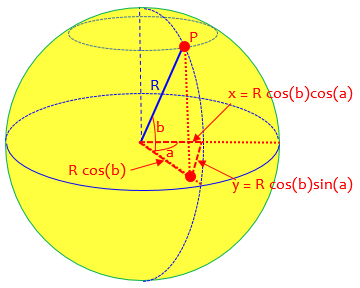Instead, we want to project points near P onto the tangent plane at P, with axes parallel to the lines of latitude and longitude at P: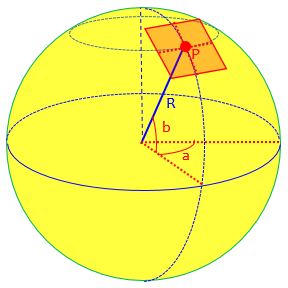I find it easiest to think a little differently. For the y coordinate, we can use the north-south distance between two lines of latitude:

y = R*(b2-b1)*pi/180

Here, I have converted the latitude difference, (b2-b1), from degrees to radians, by multiplying it by (pi radians)/(180 degrees). The product of the angle in radians and the radius is the arc length in the same units as the radius.

This will represent lines of latitude (“parallels”) as parallel horizontal lines, and vertical distances as arc lengths along lines of longitude. On the common conic projection used for small regions, lines of latitude turn out as circular arcs. We are here inventing the simplest possible local projection.

For the x coordinate, we can use the distance along a line of latitude from one line of longitude to the other:

x = R*(a2-a1)*(pi/180)*cos(b1)

Here we have an additional factor, the cosine of the latitude along which we are measuring. The line of latitude is a circle with a smaller radius than that of the equator; it is reduced by the factor cos(b1).

Lines of longitude will be represented as vertical lines (which on a conic projection would be slanted), and horizontal distances as arc lengths along the line of latitude through the first point, whose radii are $$R\cos(lon_1)$$: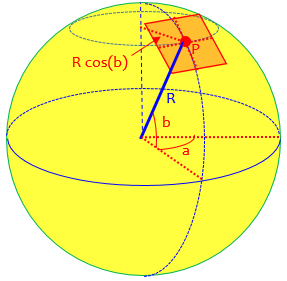Thus, you see that I have set up a coordinate system (x,y) that puts one of the points of interest at the origin. The distance from the origin to any other point (x,y) is the square root of (x^2 + y^2).


So our formula for distance from $$(lon_1,lat_1)$$ to $$(lon_2,lat_2)$$, with angles in degrees, is

$$x = \frac{\pi R}{180}(lon_2-lon_1)\cos(lat_1)\\ y = \frac{\pi R}{180}(lat_2-lat_1)\\ d = \sqrt{x^2+y^2}$$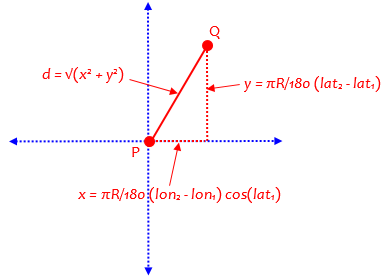### Scaling a map with a local projection

(2) We'd have to know the particular projection used in the map in order to be completely accurate: is it a Mercator projection, for instance? If the scale is small enough (as I assume based on the context of the first question), I would assume it's fairly linear over the region of the map. Then, if you want x to vary from 0 to w and y to vary from 0 to h (with the origin at the bottom left), the coordinates of a point (a,b) are

x = w*(a - a1)/(a2 - a1)
y = h*(b - b1)/(b2 - b1)

That's just a simple linear transformation. Again, it assumes that the map covers a small area. If you're asking something more complicated than this, please try again to explain it.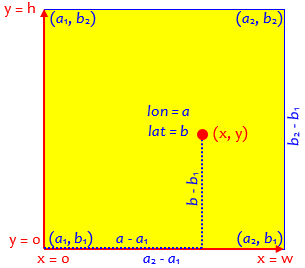Many “digital maps” use a simple version of the Mercator projection. As a conformal map, this is appropriate for use at small scales, because shapes and angles are preserved. That makes the suggestion here more or less reasonable.

### How about a few kilometers?

I'm developing a digital map that encounters the same problem but the range is bigger.  The area of the map is around 50 km^2.

Could the formulas:

x = w*(a - a1)/(a2 - a1)
y = h*(b - b1)/(b2 - b1)

be applied here?  What is the accuracy factor in this case?

In other words, can we still assume that map is linear (as if the earth were flat) for this larger scale? A square with area 50 square kilometers will have sides approximately the square root of that, namely about 7 by 7 kilometers, less than 5 miles wide and high.

Hi, Bryan.

I think a linear scaling will be fine for a map with maximum distances less than 10 miles, as your area suggests. It is hard to give a formula for the maximum error, but the following page from the Dr. Math Archives has a table that will help:

Planar Approximation: Latitude and Longitude
http://mathforum.org/library/drmath/view/62720.html


Of course, the map has to be made correctly in the first place, if we are to correctly locate points within it:

There is one assumption I didn't mention with the linear scaling formula: it assumes that the latitudes and longitudes at the corners of the map have been chosen so that the map will have the same scale horizontally and vertically. The Planar Approximation formula in the page I just mentioned will help you do this.

x = (lon2-lon1)*cos(lat1)*pi/180
y = (lat2-lat1)*pi/180

Here, x and y are in radians; if we want them in miles, we should use

x = (lon2-lon1)*cos(lat1)*pi*R/180
y = (lat2-lat1)*pi*R/180

where R is the radius of the earth, R = 6367 km = 3956 mi.

(The radian version in effect assumes a radius of 1, but takes longitude and latitude as in degrees.)

## How close is close enough?

Here is the page Doctor Rick referred to for a discussion of errors, from 2003:

Planar Approximation: Latitude and Longitude

How can I calculate the distance between two points below which they can be treated as if they were in a plane rather than on a sphere?

I am trying to calculate the midpoint between cases of legionella and their nearest neighbor.  The cases are usually within 15 miles, but how can I calculate the maximum distance at which I need to treat the two cases as if they were on a sphere (i.e. account for the curvature of the earth)?

I've seen many people mention that below 20 km or below 10 miles the earth's curvature doesn't matter - how do they calculate this?

Hi, Jessica.

It's a complicated matter. How much error the planar approximation introduces in the distance between points depends not only on the distance but on the latitude and on the direction between the points.

For instance, if one point is on the equator, distances north and south, and distances east and west, are both exact regardless of the distance (ignoring effects of nonsphericity). Errors do enter when the direction is at an angle.

At the poles, the error is extreme. In fact, if one point is at a pole, the correct formula for distance is (90-lat)*pi*R/180 where lat is the latitude of the second point and R is the radius of the earth. This is very different from the Pythagorean formula!

One issue on the equator would be the fact that the effective radius along a meridian is different from along the equator; but effects of curvature would be present even on a perfect sphere.

At the pole, with latitude 90°, our formula becomes $$x = \frac{\pi R}{180}(lon_2-lon_1)\cos(90°) = 0\\ y = \frac{\pi R}{180}(lat_2-90°)\\ d = \sqrt{x^2+y^2} = \sqrt{0^2+y^2} = |y| = \frac{\pi R}{180}(90°-lat_2)$$ which is just what he got directly. So the formula actually works, if you are exactly at the pole! Elsewhere near the pole, not so much, because lines of longitude are far from parallel. (We’ll see a better formula for that case at the end.)

The difficulty of determining when the planar approximation is safe leads to a practical consideration for programming:

If some distances will be great enough to require the spherical formula, why not just use it all the time? I know it takes more trig calculations, and if one point is fixed, the one trig function in the planar calculation can be pre-calculated, so there may be very good reasons for using the planar calculation whenever possible. But is that the case here?

I would use the Haversine formula for distance when distances may be quite small:

Deriving the Haversine Formula
http://mathforum.org/library/drmath/view/51879.html

If you'd like, I could generate a table showing the errors for various distances and directions from a given point. Let me know if this will help, and tell me what latitude to use for the reference point.

The haversine formula is what we looked at last week.

Jessica wrote back:

Thanks for your quick response Dr. Rick!  You've convinced me to use the Haversine formula.

I am programming this in SAS and need to calculate the distance 1.3 billion times in the program (this simulation is run every day too), so efficiency in calculations will factor in.  All of the cases are in NYC (Lat range is 40.5 to ~41 dd and Long is -73.7 to -74.3 dd).  I think an error table will be very useful; thank you for the offer.

I have been using the law of cosines for spherical trig formula to calculate the distance between two points, but will replace it with the Haversine formula for all distance calculations.


I am omitting a further question about finding the midpoint between two points, which I may post in the future.

Doctor Rick replied, starting with more advice on efficiency:

Since you need an efficient algorithm and you are (in the first phase) only interested in *comparing* distances, I suggest that you only calculate 'a' in the Haversine algorithm. It is the square of half the chord length between the two points, so of two choices for point B, the one that has a smaller 'a' value is closer to point A. This saves you two square roots and an atan2 for each point in your search. Of course, you can pre-calculate cos(lat1) for the given point A, leaving only three trig calculations per point in the search.

It is also possible that the huge number of calculations needed could be reduced by changes at a higher level in the program.

For the error table: I set up a spreadsheet to compute a table of relative errors due to the planar approximation for points at various distances and bearings. The table has a column for each distance (in kilometers) and a row for each bearing (in degrees from north), from a point at a given latitude (41 degrees). For each distance and bearing, I computed the latitude and longitude of the second point using the exact spherical formula:

LAT, LON GIVEN DISTANCE (d*R) AND AZIMUTH (tc)
lat2=asin(sin(lat1)*cos(d)+cos(lat1)*sin(d)*cos(tc))
IF (cos(lat)=0)
lon2=lon1      // endpoint a pole
ELSE
lon2=mod(lon1-asin(sin(tc)*sin(d)/cos(lat1))+pi,2*pi)-pi
ENDIF

Then I computed the distance using the approximate formula:

PLANAR APPROXIMATION
x = (lon2-lon1)*cos(lat1)*pi/180
y = (lat2-lat1)*pi/180
d = R * sqrt(x^2 + y^2)

This formula for finding a point given distance and bearing is from Doctor Rick’s answer in

Finding Points on the Earth

He got it, in turn, from a highly-recommended source:

Aviation Formulary V1.30 – Ed Williams

The distances I found were:

CALCULATED DISTANCE BY PLANAR APPROXIMATION
distance (km)
1            2            5            10           50
0  1            2            5            10           50
15  1.000004416  2.000017668  5.000110532  10.00044283  50.01121206
30  1.000014774  2.000059113  5.000369752  10.00148097  50.03741972
45  1.000024125  2.000096516  5.000603557  10.00241644  50.06085552
60  1.000025585  2.000102347  5.000639801  10.00256008  50.06417495
75  1.000016472  2.000065881  5.000411598  10.00164534  50.0409209
90  0.999999996  1.999999969  4.999999515   9.999996121 49.99951521
105  0.999983524  1.999934086  4.999587879   9.998350475 49.95855614
120  0.999974418  1.999897681  4.999360636   9.997443424 49.9362629
135  0.999975884  1.999903555  4.999397548   9.997592399 49.94024953
150  0.999985233  1.999940949  4.999631225   9.998526849 49.96355763
165  0.999995587  1.999982354  4.999889816   9.99955996  49.98913625
180  1            2            5            10           50


For example, the point 50 km away, 60° east of north, was found to be 50.06417495 km away by the planar approximation, only about 64 meters too far.

Finally I computed the relative error, that is,

(approx. distance - actual distance)/(actual distance)

RELATIVE ERROR
distance (km)
1            2            5           10           50
0  1.40554E-13   5.3535E-13   1.4051E-13  -1.74083E-14  1.42109E-14
15  4.41569E-06   8.83417E-06  2.21064E-05  4.42826E-05  0.000224241
30  1.47744E-05   2.95567E-05  7.39505E-05  0.000148097  0.000748394
45  2.41246E-05   4.8258E-05   0.000120711  0.000241644  0.00121711
60  2.55851E-05   5.11736E-05  0.00012796   0.000256008  0.001283499
75  1.64723E-05   3.29404E-05  8.23196E-05  0.000164534  0.000818418
90 -3.87847E-09  -1.55143E-08 -9.6964E-08  -3.87854E-07 -9.69588E-06
105 -1.64765E-05  -3.29571E-05 -8.24242E-05 -0.000164952 -0.000828877
120 -2.55816E-05  -5.11596E-05 -0.000127873 -0.000255658 -0.001274742
135 -2.41158E-05  -4.82227E-05 -0.00012049  -0.00024076  -0.001195009
150 -1.47666E-05  -2.95254E-05 -7.3755E-05  -0.000147315 -0.000728847
165 -4.41291E-06  -8.82303E-06 -2.20367E-05 -4.4004E-05  -0.000217275
180  1.40554E-13   1.40554E-13  1.4051E-13  -1.74083E-14  1.42109E-14

You can see that, at latitude 41 degrees, the greatest error at 10 km is 0.0256%, or 2.56 m. Not bad, I'd say. At 50 km the greatest error is 0.128%, or 64 m. That's better than I expected, frankly. At 100 km the error is still under 258 meters (0.26%).

The claim that 20 km is close enough to ignore curvature seems to be true – though it still depends on the accuracy you need.

### Explaining the planar approximation

Many thanks to you!  That was brilliant and very useful!

One final question - why is the cos(lat1) term added to the x term?

PLANAR APPROXIMATION
x = (lon2-lon1)*cos(lat1)*pi/180
y = (lat2-lat1)*pi/180
d = R * sqrt(x^2 + y^2)

This essentially asks for more detail on the original derivation. Doctor Rick answered,

Hi, Jessica.

The lines of longitude get closer together as they head toward the poles. Therefore a degree of longitude corresponds to a shorter distance as the latitude increases.

To make this quantitative, consider a line of latitude, which is a circle. Looking at a side view of the earth (a cross-section along a line of longitude), we see that the radius of the latitude circle, r, and the radius of the earth, R, form a right triangle with an angle that is the latitude:

*********
******    |    ******
***          +----------***
**             |     r      /**
**               |           / | **
**                 |          /  |   **
*                   |         /   |     *
*                    |        /    |      *
*                     |       /     |       *
*                      |      /R     |        *
*                       |     /       |         *
*                        |    /        |          *
*                        |   /         |          *
*                         |  /          |           *
*                         | /           |           *
*                         |/ lat        |           *
*-------------------------*-------------+-----------*
r

Thus we see that cos(lat) = r/R, so r = R*cos(lat).

So that’s the radius of the line of latitude.

Now, the difference in longitude between two points at the same latitude is a central angle of the latitude circle. The distance between the points is the radius of the circle times the angle in radians. To convert the longitude difference to radians, we multiply it by pi/180. Then we multiply by r = R*cos(lat) to get the distance x:

x = (lon2-lon1)*(pi/180)*R*cos(lat)

Since we are using the latitude of the first point, we would get different distances if we swapped the two points!

## A polar flat earth approximation

In preparing last week’s post, I found that the GIS FAQ used as a source there also included a “flat-earth approximation” that was different from Doctor Rick’s; and when I used it for my sample distance between two points in Paris, I got a considerably different answer (2.28 rather than 2.12 km)! I only realized later that they don’t claim that formula applies everywhere. Let’s take a look.

If the distance is less than about 20 km (12 mi) and the locations of the two points in Cartesian coordinates are X1,Y1 and X2,Y2 then the

Pythagorean Theorem

d = sqrt((X2 - X1)^2 + (Y2 - Y1)^2)

will result in an error of
less than 30 meters (100 ft) for latitudes less than 70 degrees
less than 20 meters ( 66 ft) for latitudes less than 50 degrees
less than  9 meters ( 30 ft) for latitudes less than 30 degrees
(These error statements reflect both the convergence of the meridians and the curvature of the parallels.)

The flat-Earth distance d will be expressed in the same units as the coordinates.

If the locations are not already in Cartesian coordinates, the computational cost of converting from spherical coordinates and then using the flat-Earth model may exceed that of using the more accurate spherical model.

They have not actually stated how to convert to Cartesian coordinates; it is possible that they intended to use something like Doctor Rick’s formula. In any case, they, too, recommend using the haversine formula instead.

Later, they give another formula that is meant only for locations close to the poles, where they pretend that the entire polar region is flat, and apply the Law of Cosines to a triangle with one vertex at the pole: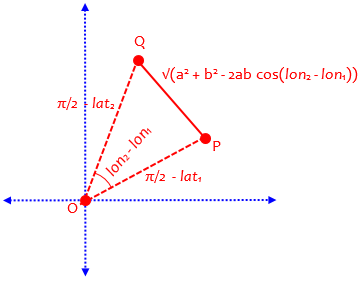The Pythagorean flat-Earth approximation assumes that meridians are parallel, that the parallels of latitude are negligibly different from great circles, and that great circles are negligibly different from straight lines.  Close to the poles, the parallels of latitude are not only shorter than great circles, but indispensably curved.  Taking this into account leads to the use of polar coordinates and the planar law of cosines for computing short distances near the poles: The

Polar Coordinate Flat-Earth Formula

a = pi/2 - lat1
b = pi/2 - lat2
c = sqrt(a^2 + b^2 - 2 * a * b * cos(lon2 - lon1)
d = R * c

will give smaller maximum errors than the Pythagorean Theorem for higher latitudes and greater distances.  (The maximum errors, which depend upon azimuth in addition to separation distance, are equal at 80 degrees latitude when the separation is 33 km (20 mi), 82 degrees at 18 km (11 mi), 84 degrees at 9 km (5.4 mi).)  But even at 88 degrees the polar error can be as large as 20 meters (66 ft) when the distance between the points is 20 km (12 mi).

The latitudes lat1 and lat2 must be expressed in radians (see above); pi/2 = 1.5707963.  Again, the intermediate result c is the distance in radians and the distance d is in the same units as R.

This site uses Akismet to reduce spam. Learn how your comment data is processed.